Case Based Questions (MCQ)

Chapter 12 Class 10 Areas related to Circles
Serial order wise
This question is inspired from Ex 12.2, 8 - Chapter 12 Class 10 - Areas Related to Circles

## A horse is tied to a peg at one corner of a square  shaped grass field of sides 15 m by means of a 5 m  long rope (see the given figure).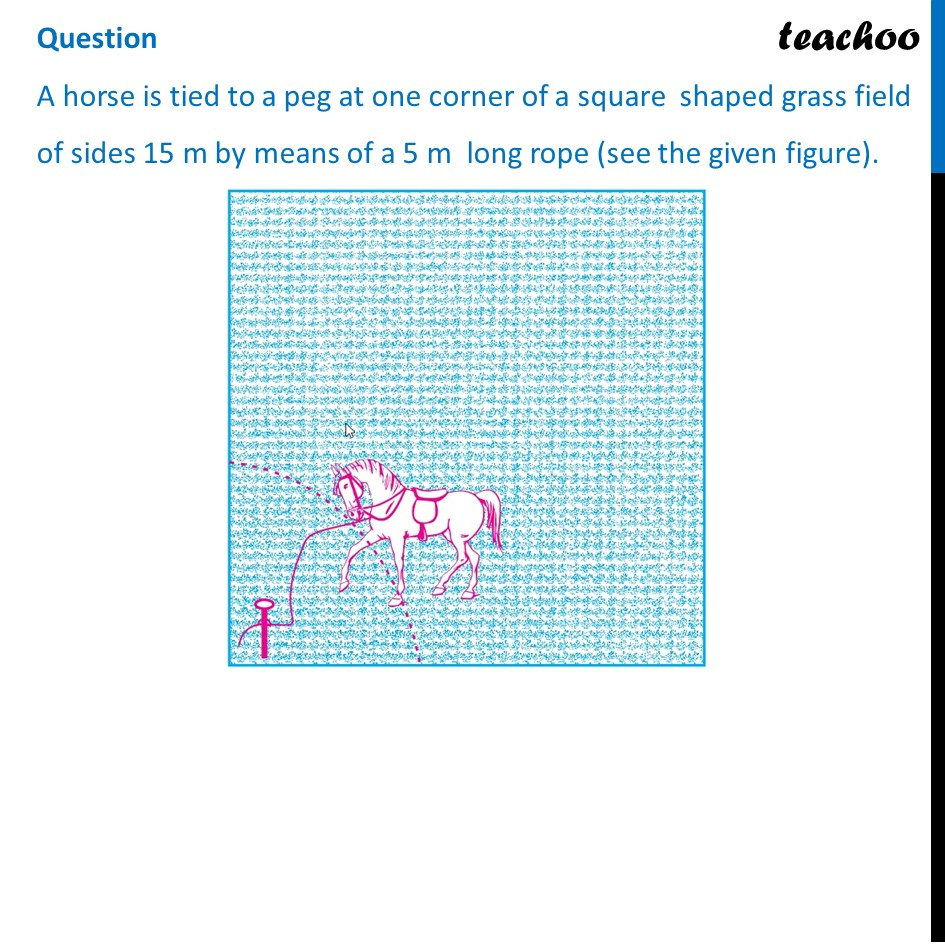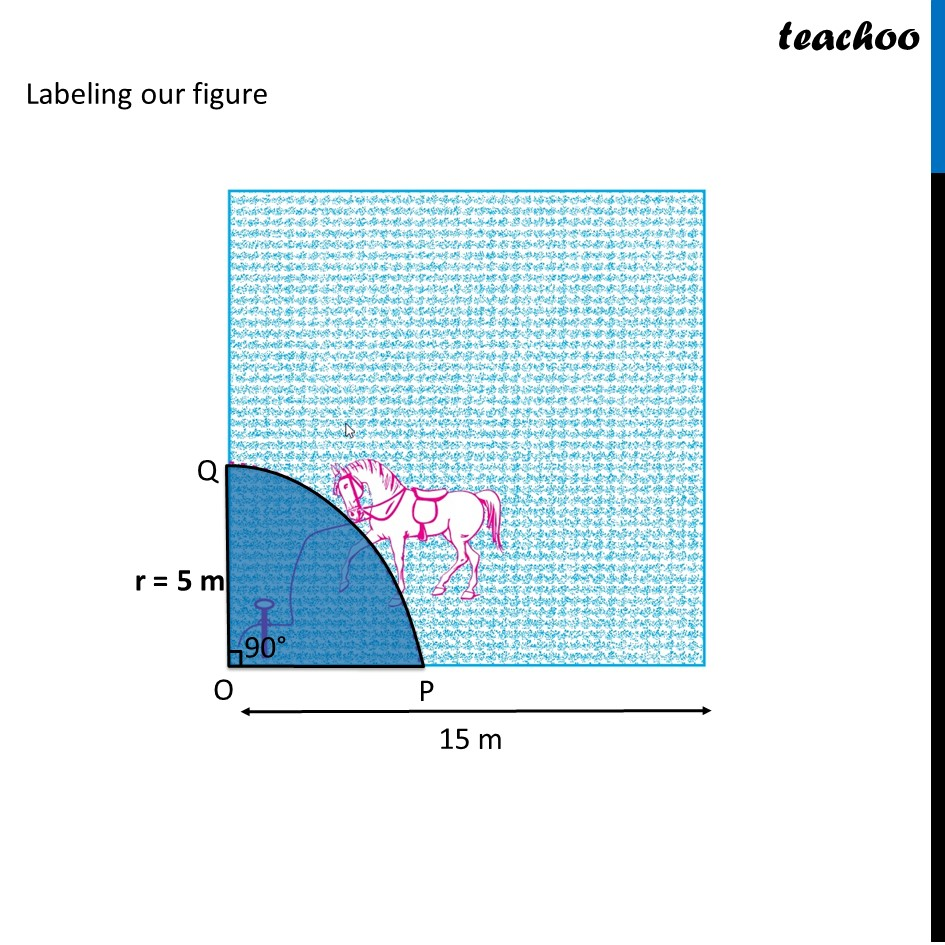## (c) 255 m 2                   (d) 15 m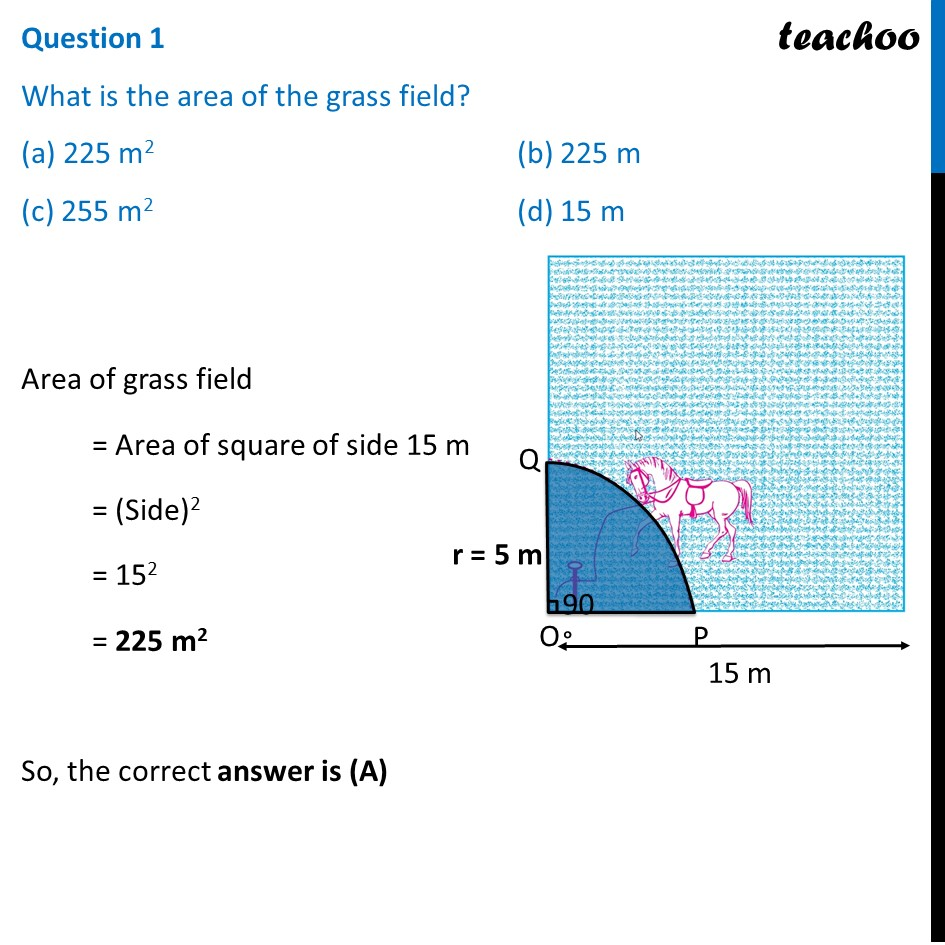## (c) 19 m 2   (d) 78.5 m 2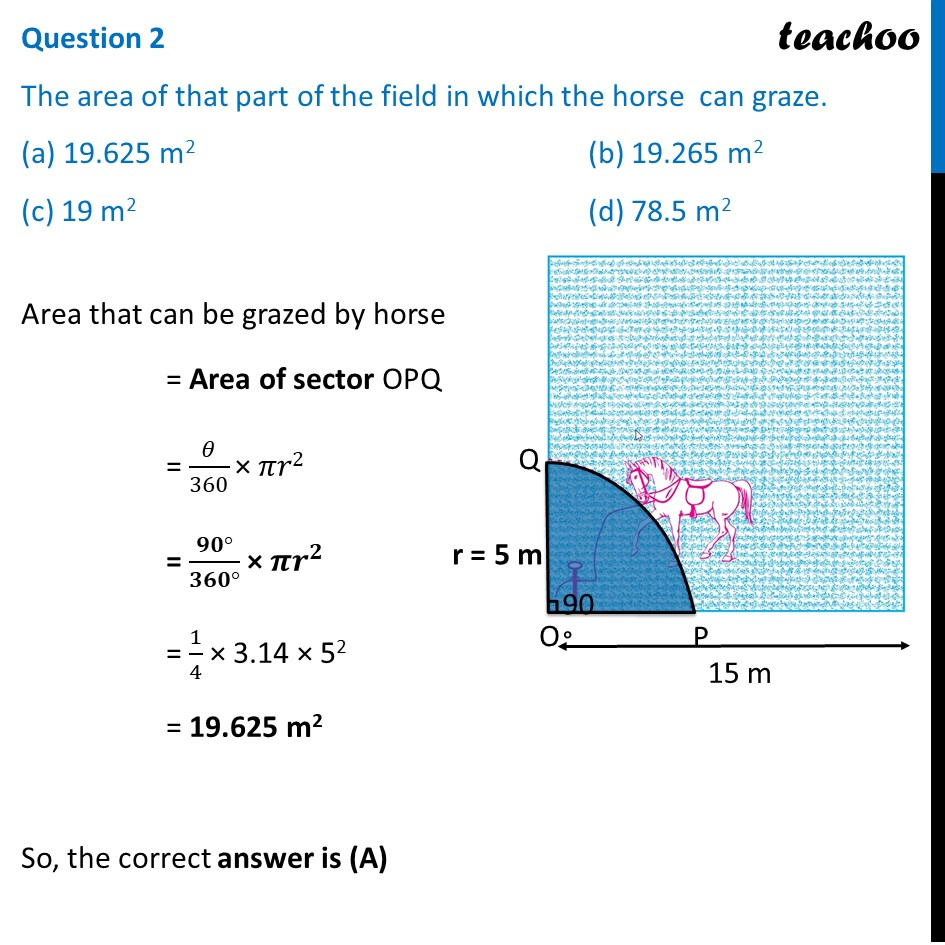## (c) 225 m 2                                    (d) 78.5 m 2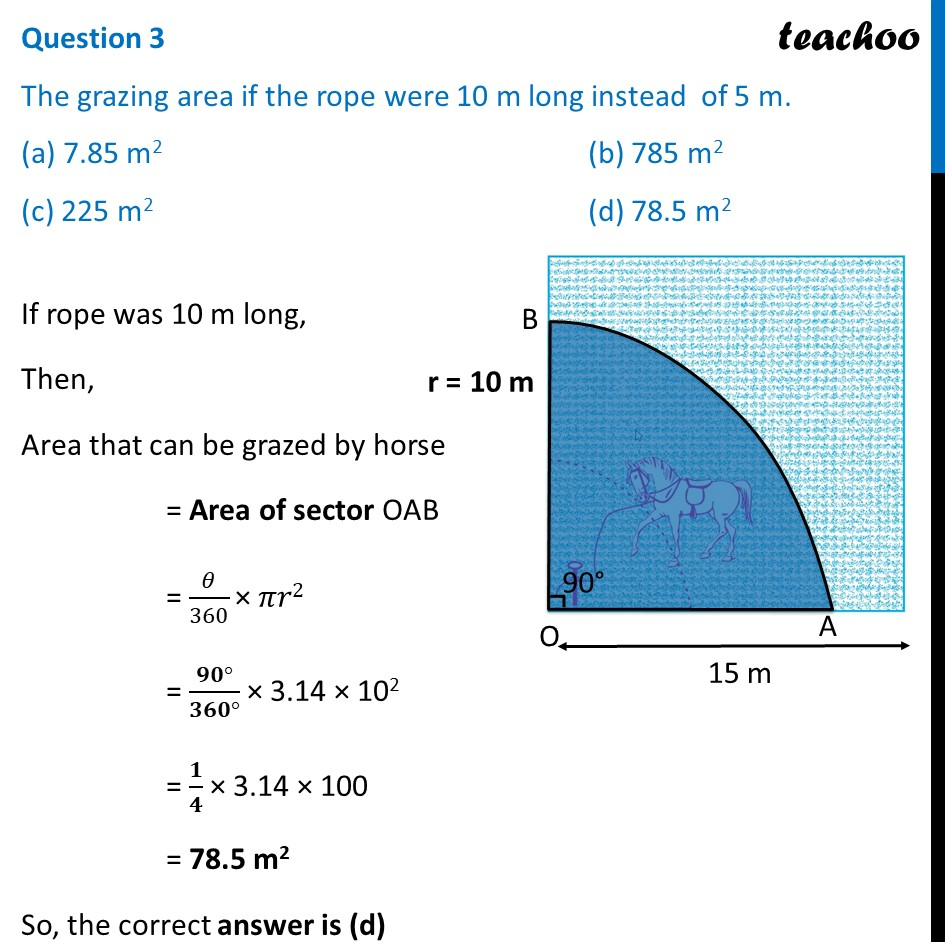## (c) 58 m 2                                           (d) 78.5 m 2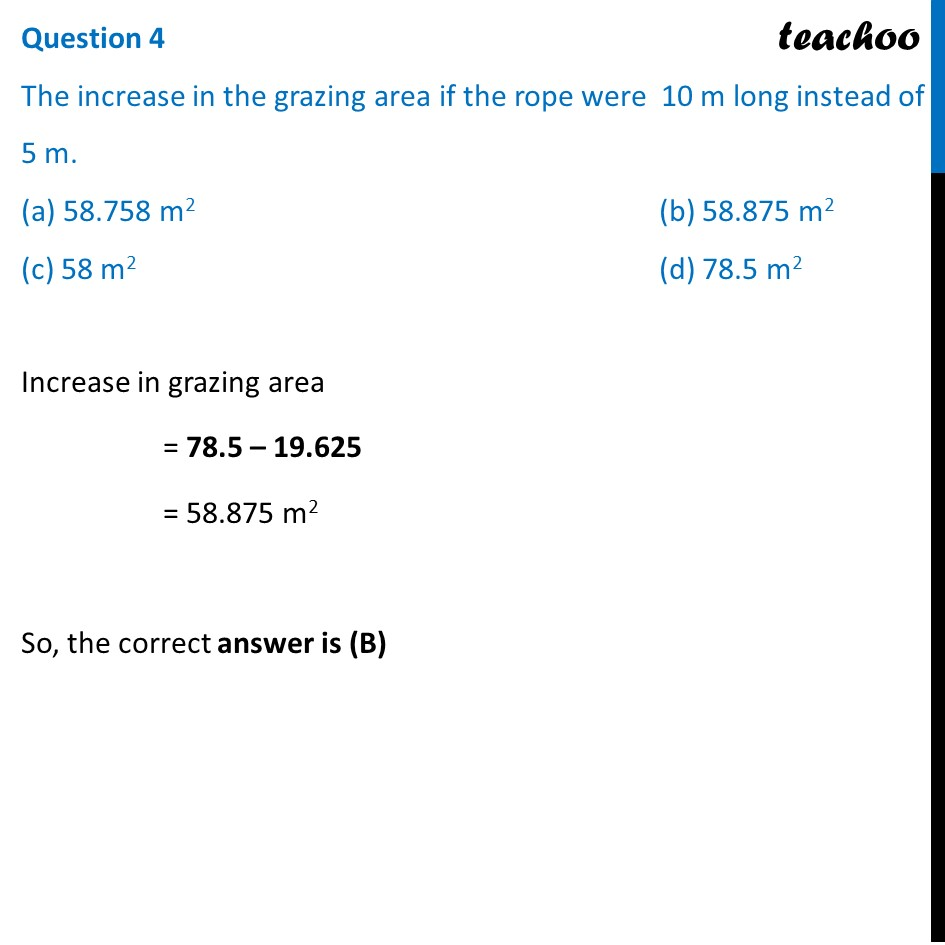## (c) Circle                                      (d) None of these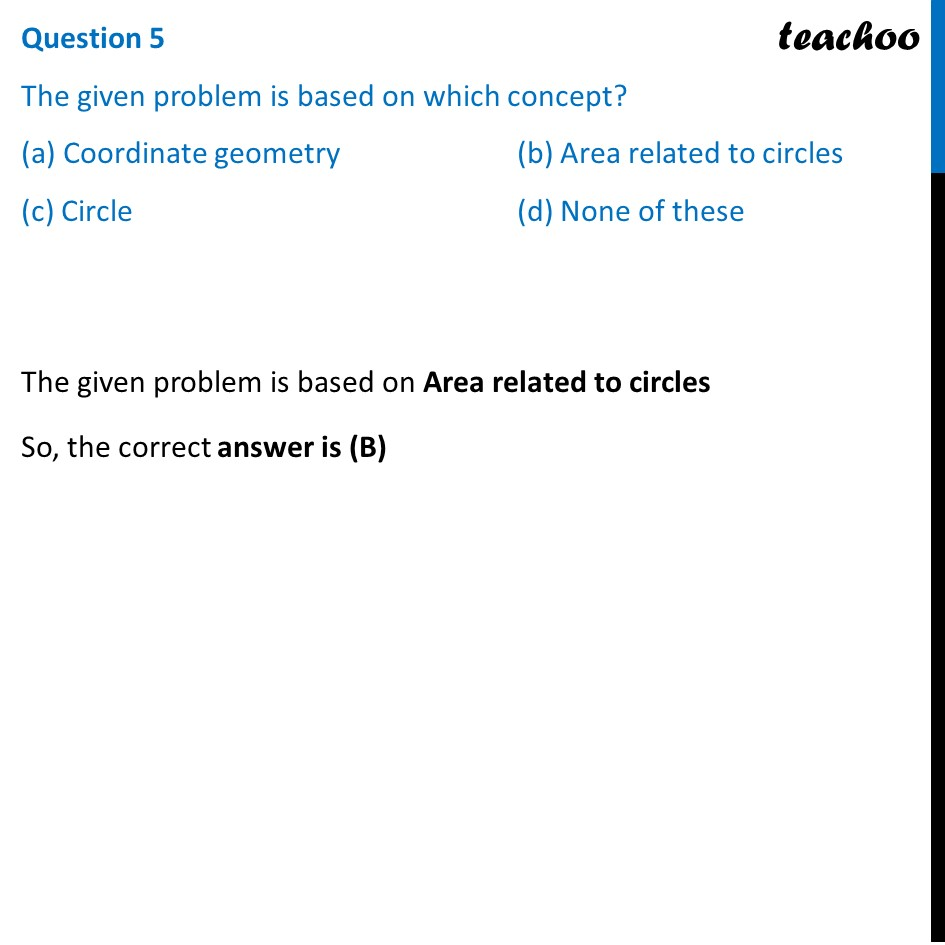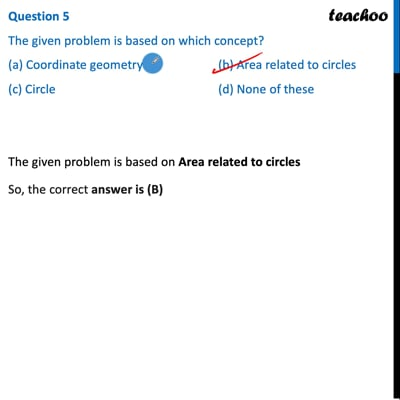This video is only available for Teachoo black users

Maths Crash Course - Live lectures + all videos + Real time Doubt solving!

### Transcript

Question A horse is tied to a peg at one corner of a square shaped grass field of sides 15 m by means of a 5 m long rope (see the given figure). Labeling our figure Question 1 What is the area of the grass field? (a) 225 m2 (b) 225 m (c) 255 m2 (d) 15 m Area of grass field = Area of square of side 15 m = (Side)2 = 152 = 225 m2 So, the correct answer is (A) Question 2 The area of that part of the field in which the horse can graze. (a) 19.625 m2 (b) 19.265 m2 (c) 19 m2 (d) 78.5 m2 Area that can be grazed by horse = Area of sector OPQ = 𝜃/360 "×" 𝜋𝑟2 = (𝟗𝟎°)/(𝟑𝟔𝟎°) × 𝝅𝒓^𝟐 = 1/4 × 3.14 × 52 = 19.625 m2 So, the correct answer is (A) Question 3 The grazing area if the rope were 10 m long instead of 5 m. (a) 7.85 m2 (b) 785 m2 (c) 225 m2 (d) 78.5 m2 If rope was 10 m long, Then, Area that can be grazed by horse = Area of sector OAB = 𝜃/360 "×" 𝜋𝑟2 = (𝟗𝟎°)/(𝟑𝟔𝟎°) × 3.14 × 102 = 𝟏/𝟒 × 3.14 × 100 = 78.5 m2 So, the correct answer is (d) Question 4 The increase in the grazing area if the rope were 10 m long instead of 5 m. (a) 58.758 m2 (b) 58.875 m2 (c) 58 m2 (d) 78.5 m2 Increase in grazing area = 78.5 – 19.625 = 58.875 m2 So, the correct answer is (B) Question 5 The given problem is based on which concept? (a) Coordinate geometry (b) Area related to circles (c) Circle (d) None of these The given problem is based on Area related to circles So, the correct answer is (B)